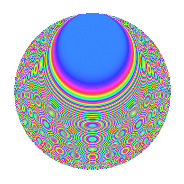# Properties

 Label 2016.2.bzLevel 2016 Weight 2 Character orbit bz Rep. character $$\chi_{2016}(239,\cdot)$$ Character field $$\Q(\zeta_{6})$$ Dimension 144 Sturm bound 768

# Related objects

## Defining parameters

 Level: $$N$$ = $$2016 = 2^{5} \cdot 3^{2} \cdot 7$$ Weight: $$k$$ = $$2$$ Character orbit: $$[\chi]$$ = 2016.bz (of order $$6$$ and degree $$2$$) Character conductor: $$\operatorname{cond}(\chi)$$ = $$72$$ Character field: $$\Q(\zeta_{6})$$ Sturm bound: $$768$$

## Dimensions

The following table gives the dimensions of various subspaces of $$M_{2}(2016, [\chi])$$.

Total New Old
Modular forms 800 144 656
Cusp forms 736 144 592
Eisenstein series 64 0 64

## Trace form

 $$144q + O(q^{10})$$ $$144q - 72q^{25} - 24q^{27} - 16q^{33} + 24q^{41} + 72q^{49} + 8q^{57} + 72q^{59} + 112q^{75} - 8q^{81} + 64q^{99} + O(q^{100})$$

## Decomposition of $$S_{2}^{\mathrm{new}}(2016, [\chi])$$ into newform subspaces

The newforms in this space have not yet been added to the LMFDB.

## Decomposition of $$S_{2}^{\mathrm{old}}(2016, [\chi])$$ into lower level spaces

$$S_{2}^{\mathrm{old}}(2016, [\chi]) \cong$$ $$S_{2}^{\mathrm{new}}(72, [\chi])$$$$^{\oplus 6}$$$$\oplus$$$$S_{2}^{\mathrm{new}}(288, [\chi])$$$$^{\oplus 2}$$$$\oplus$$$$S_{2}^{\mathrm{new}}(504, [\chi])$$$$^{\oplus 3}$$

## Hecke Characteristic Polynomials

There are no characteristic polynomials of Hecke operators in the database